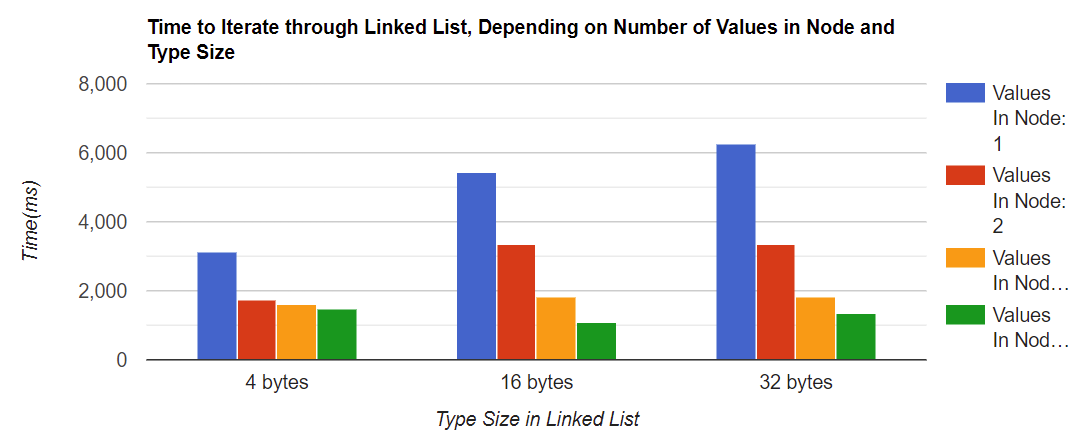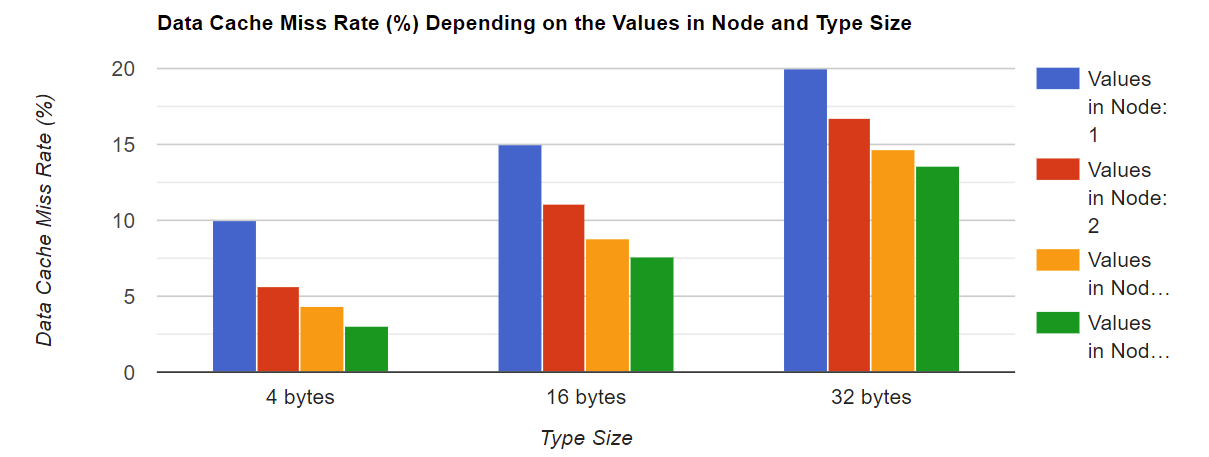# 让程序运行更快的一些技巧

## 经常访问的数据，在内存中应当是相邻的

 class free_memory_list {
void* head; /// Pointer to the beginning of the list
Statistics statistics; /// Statistics about list usage
int count; /// Number of elements in the list
Allocator* base_allocator; /// Pointer to the class used for memory
/// allocation and deallocation
};


## 优化对类或结构数组的访问

• 类的大小需要是cache line大小的倍数。
• 数组的起始地址需要是cache line大小的倍数。

my_class* array_of_my_class;
posix_memalign((void**)array_of_my_class, 64, SIZE * sizeof(my_class));
for (size_t i = 0; i < SIZE; i++) {
::new (&array_of_my_class[i]) my_class(i);
}


## 有效地访问你的矩阵中的数据

void multiply_matrices(int in_matrix1[][N], int in_matrix[][N], int result[][N])
{
int i, j, k;
for (i = 0; i < N; i++) {
for (j = 0; j < N; j++) {
result[i][j] = 0;
for (k = 0; k < N; k++) {
result[i][j] += in_matrix1[i][k] * in_matrix[k][j];
}
}
}
}


void multiply_matrices(int in_matrix1[][N], int in_matrix[][N], int result[][N])
{
int i, j, k;
for (i = 0; i < N; i++) {
for (j = 0; j < N; j++) {
result[i][j] = 0;
}
for (k = 0; k < N; k++) {
for (j = 0; j < N; j++) {
result[i][j] += in_matrix1[i][k] * in_matrix[k][j];
}
}
}



## 在你的类和结构体中避免填充

class my_class {
int my_int;
double my_double;
int my_second_int;
};


class my_class {
double my_double;
int my_int;
int my_second_int;
};


## 尽可能的使用最小的类型

class my_class {
public:
bool my_bool1:1;
bool my_bool2:1;
bool my_bool3:1;
bool my_bool4:1;
int my_int:1;
};


## 尽可能避免堆分配

• 调用 mallocfree 是很慢的。
• 对这些位置的访问是间接(通过指针)的，这种方式将导致更多的高速缓存不命中的情况。

## 尽可能的重复使用缓冲中的数据

int * a = initialize_array(size);
int min = find_min(a, size);
int max = find_max(a, size);


int * a = initialize_array(size);
int min = a;
int max = a;
for (int i = 0; i < size; i++) {
min = std::min(a[i], min);
max = std::max(a[i], max);
}


## 尽量避免写内存

void sort_fast(int* a, int len) {
for (int i = 0; i < len; i++) {
int min = a[i];
int min_index = i;
for (int j = i+1; j < len; j++) {
if (a[j] < min) {
min = a[j];
min_index = j;
}
}
std::swap(a[i], a[min_index]);
}
}
void sort_slow(int* a, int len) {
for (int i = 0; i < len; i++) {
for (int j = i+1; j < len; j++) {
if (a[j] < a[i]) {
std::swap(a[j], a[i]);
}
}
}
}


## 正确对齐你的数据

unsigned char serialized_data;
int* header_pointer = (int*) (serialized_data + 3);


unsigned char serialized_data;
int* header_pointer = (int*) (serialized_data + 3);


## 使用软件预取

int binarySearch(int *array, int number_of_elements, int key) {
int low = 0, high = number_of_elements-1, mid;
while(low <= high) {
mid = (low + high)/2;
#ifdef DO_PREFETCH
// low path
__builtin_prefetch (&array[(mid + 1 + high)/2], 0, 1);
// high path
__builtin_prefetch (&array[(low + mid - 1)/2], 0, 1);
#endif
if(array[mid] < key)
low = mid + 1;
else if(array[mid] == key)
return mid;
else if(array[mid] > key)
high = mid-1;
}
}


# 实验

## 带软件预取的二分搜索算法

 for (int i = 0; i < len; i++) {
binarySearch(inputArray, len, inputArray[indexArray[i]]);
}


10K 1673ms 1777ms -6.2%
100K 2478ms 2426ms +2.1%
1M 4519ms 3996ms +11.6%
10M 8804ms 7096ms +19.4%
100M 14970ms 11685ms +21.9%

10K 977ms 1168ms -19.5%
100K 1122ms 1380ms -23.0%
1M 1367ms 1623ms -18.7%
10M 1610ms 1892ms -17.5%
100M 1813ms 2171ms -19.7%

10K 1112ms 1418ms -27.5%
100K 1766ms 1942ms -9.7%
1M 3018ms 2792ms +7.5%
10M 3236ms 3019ms +6.7%
100M 3356ms 3182ms +5.2%

10K 760ms 984ms -29.5%
100K 1049ms 1508ms -43.8%
1M 2739ms 2640ms +3.6%
10M 4402ms 7490ms -70.1%
100M 10395ms 8251ms -20.6%

Parameter 关闭Prefetching 开启Prefetching 差异

Cycles 31481M 25648M -18.5%

## 缓存友好的链表

    class linked_list_node {
public:
char used_elems[count];
char values[count * sizeof(Type)];
...
};# 参考

Ulrich Drepper “What every programmer should know about memory”

What is cache memory – Gary explains

Agner.org – Software Optimization Resources

posted @ 2022-03-20 13:32  PcDack  阅读(274)  评论(0编辑  收藏  举报